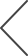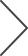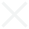search.noResults

search.searchingnote.createNoteMessagesearch.noResults

search.searching

#### orderForm.title

orderForm.productCode
orderForm.description
orderForm.quantity
orderForm.itemPrice
orderForm.price
orderForm.totalPrice
orderForm.noItems
3D Fourier Transform Analysis

In the case of the tilted-beam

Figure 3 : Schematics showing the 3D FT analysis in the case of the normal illumination. (a) I xyz : stack of acquired through-focus TEM images. (b) I uvz : stack of the 2D FT (diffractogram) of each image. (c) I uvw : 3D FT of the image stack. The 3D FT shows two Ewald spheres that attach at the origin and are rotationally symmetric around the w -axis. The information limit can be estimated from the fading of the 3D FT intensity on the Ewald spheres as indicated by a vertical broken line in (c). In the case of a tilted-beam illumination, the two Ewald spheres slant as shown in Figures 4 and 5 .

envelopes E t assuming beam-tilt angles of 1, 3, and 5 degrees for 80 kV. Here, we suppose two defocus spreads ∆ of 5.0 nm and 0.5 nm for (a) and (b), respectively, where the square box in (b) corresponds to the whole area of (a). T e circle (or the arc) in each fi gure indicates the position of the achromatic circle. T e temporal envelope is sharp for a large beam tilt. However, it becomes broad for a small defocus spread as shown in (b).

Now, we consider the diff ractogram D , which is the squared modulus of 2D FT:

×

where we neglect the non-linear term in Eq. (1) and include the rotationally symmetric dumping function M , such as the modulation transfer function (MTF) of the recording system. Here, we assume the relation

(that is,

kinematical scattering) for the scattered wave, which restricts applicability of the diffractogram analysis only to a weak scattering sample. In the case of a C s -corrected microscope, since the information limit is mainly determined by the temporal envelope as described above, the diffractogram may be approximated by

×

where the last term describes interference between two linear terms. In this equation Et() and

respectively denote the

temporal envelope and the wave aberration function for the beam-tilt directions

illumination becomes

or . T e diff ractogram for the normal ,

where sinusoidal oscillations are known as T on’s rings [ 6 ]. We note that an absolute measurement of the temporal envelope from the normal-beam diff ractogram is diffi cult since it requires information on the rotationally symmetric dumping factors such as the MTF, M ( g ), and the scattering behavior of the sample,

. 2018 March • www.microscopy-today.com 45 where ×

diff ractogram, we can measure the rotationally symmetric factors using the fact that the temporal envelope has no attenuation on the achromatic circle. In addition, oscillations (T on’s rings) of the diff ractogram may be washed out by applying a low-pass fi lter [ 1 ] or may be ignored by consid- ering the envelope of the oscillations [ 2 ]. Nevertheless, even such tilted- beam diff ractogram analysis may become diffi cult for evaluating a C s -corrected microscope with a C c -corrector or a monocromator since the temporal envelope becomes broad for a small defocus spread. As noted before an analysis of the temporal envelope at high-angle requires a

strong scattering sample, which will inevitability introduce the strong non-linear term in the diff ractogram. However, diff rac- togram analysis cannot be applied to such a sample since it does not distinguish between linear and non-linear contributions, contrary to the 3D FT analysis discussed in the next section. 3D FT analysis . Figure 3 schematically shows the 3D FT analysis in the case of the normal illumination. Figure 3a is a stack of through-focus images, I xyz , as a function of the defocus z, while Figure 3c is the 3D FT of the image stack, I uvw , which shows two spheres called Ewald spheres. T e fi gure in the middle ( Figure 3b ) shows a stack of the diff ractograms (2D FT), I uvz , of each image intensity. We note that the information limit can be roughly estimated from an observable range of the Ewald spheres as indicated in Figure 3c . However, the intensity on the Ewald sphere is aff ected also by the rotationally symmetric attenuation factors as in the case of the diff ractogram. T erefore, a quantitative evaluation of the temporal envelope requires a 3D FT analysis of tilted-beam through-focus images. In practice a 3D FT is calculated by taking a 3D FT of the image stack in a single step. Here, however, we evaluate a 3D FT in two steps, namely by taking a 2D FT of each image of the image stack to give I uvz and then taking a 1D FT of I uvz along z -axis. T is will make clear the relation between the 2D FT (diff ractogram) and the 3D FT. We note that the 2D FT of a tilted-beam image (Eq. 1) depends on z only through the 2D TCC (Eq. 2), which in turn depends on the defocus term of the wave aberration function spatial envelope

. We have neglected the for simplicity. For a complete

analysis, please see [ 7 ]. T us, the 3D FT of the image stack may be written by replacing T 2 in Eq. (1) with the 3D TCC T 3 , which is a FT of the 2D TCC along the z-direction:

Page 1  |  Page 2  |  Page 3  |  Page 4  |  Page 5  |  Page 6  |  Page 7  |  Page 8  |  Page 9  |  Page 10  |  Page 11  |  Page 12  |  Page 13  |  Page 14  |  Page 15  |  Page 16  |  Page 17  |  Page 18  |  Page 19  |  Page 20  |  Page 21  |  Page 22  |  Page 23  |  Page 24  |  Page 25  |  Page 26  |  Page 27  |  Page 28  |  Page 29  |  Page 30  |  Page 31  |  Page 32  |  Page 33  |  Page 34  |  Page 35  |  Page 36  |  Page 37  |  Page 38  |  Page 39  |  Page 40  |  Page 41  |  Page 42  |  Page 43  |  Page 44  |  Page 45  |  Page 46  |  Page 47  |  Page 48  |  Page 49  |  Page 50  |  Page 51  |  Page 52  |  Page 53  |  Page 54  |  Page 55  |  Page 56  |  Page 57  |  Page 58  |  Page 59  |  Page 60  |  Page 61  |  Page 62  |  Page 63  |  Page 64  |  Page 65  |  Page 66  |  Page 67  |  Page 68  |  Page 69  |  Page 70  |  Page 71  |  Page 72  |  Page 73  |  Page 74  |  Page 75  |  Page 76